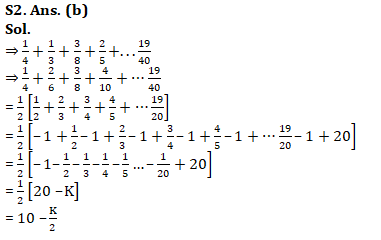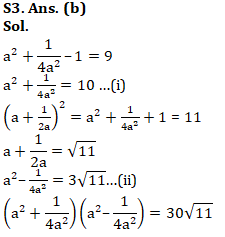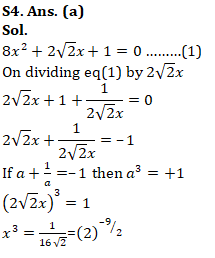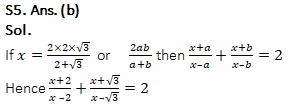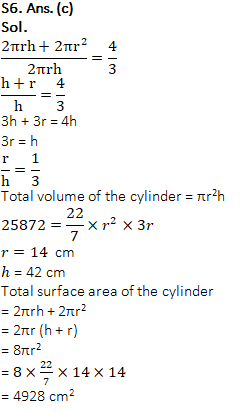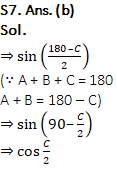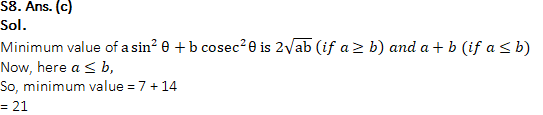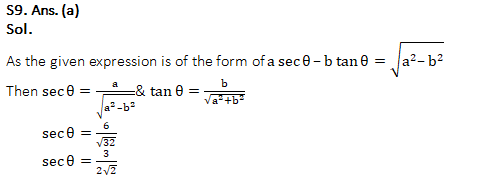# Quantitative Aptitude of Advanced Level For SSC CGL : 16th January 2020 for Number system, mensuration and Algebra

Q1. PQRS is a rectangle in which sides PQ = 24 cm and QR = 10 cm and T is a point on line RS. What is the ratio of the area of ∆TUQ and ∆QRT if PU : UQ = 1 : 2 ?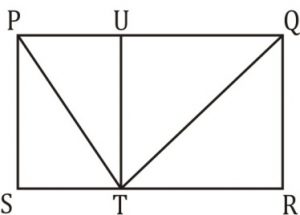(a) 8 : 7
(b)1 : 1
(c)cannot be determined
(d)4:3

Q2.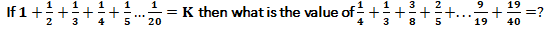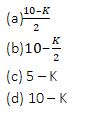Q3.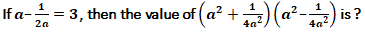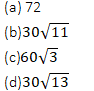Q4.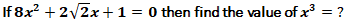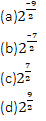Q5.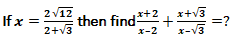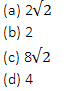Q6. A right circular cylinder is formed. Ratio of total surface area of the cylinder to its total curved surface area is 4 : 3. If volume of the cylinder is 25872 cm3 then find the total surface area(in cm2) of cylinder ?
(a) 3696
(b) 6160
(c) 4928
(d) 7392

Q7.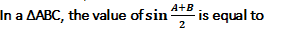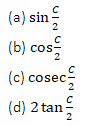Q8. What is the minimum value of function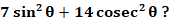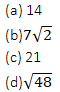Q9.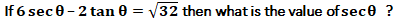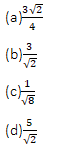Q10.The maximum length of a pencil that can be kept in a rectangular box of dimensions 8 cm × 6 cm × 2 cm, is
(a) 2 √13 cm
(b) 2 √14 cm
(c) 2 √26 cm
(d) 10 √2 cm

Solutions:

S1. Ans. (c)
Sol.
As area of ∆QTR cannot be determined hence the required ratio cannot be determined.